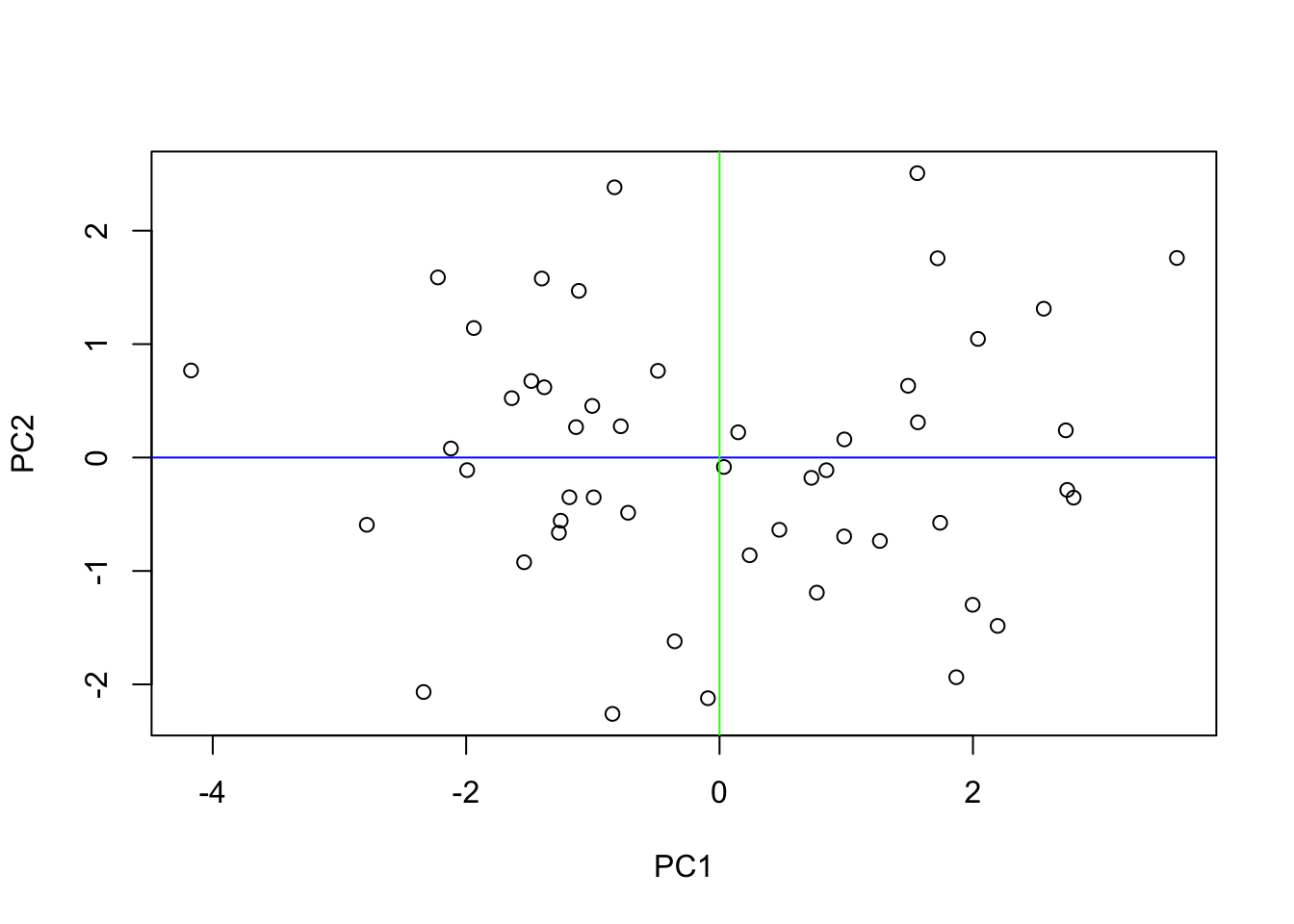In the previous sections we discussed the computation of principal components and how to pick a reasonable number of principal components to represent the data. In this section we will learn how to project the original data onto a new feature space with less dimensions. Recall that the principal components are the eigenvectors of the covariance matrix and that the eigenvalues correspond to the explained variance of the respective principal component.

In PCA the elements of the principal components are denoted as loadings ($$\phi$$). Written in vector form the loadings are referred to as loading vector, $$\mathbf p_j$$, where $$j=1,2,...,k$$.

Let us take a look at the loading of the first two principal components, $$p_1$$ and $$p_2$$, of the food-texture data set.

# load data from previous sections

food.pca.eigen <- eigen(cov(food.pca))
pca.loading
##                 PC1        PC2
## Oil       0.4575334  0.3704389
## Density  -0.4787455 -0.3567500
## Crispy    0.5323877 -0.1976610
## Fracture -0.5044769  0.2212399
## Hardness  0.1534026 -0.8046661

The output from R can be rewritten in vector form.

\begin{align} \mathbf p_1= \begin{bmatrix} \phi_{11}\\ \phi_{12}\\ \phi_{13}\\ \phi_{14}\\ \phi_{15}\\ \end{bmatrix} = \begin{bmatrix} 0.46 \\ -0.48 \\ 0.53 \\ -0.5 \\ 0.15 \\ \end{bmatrix}\text{,} \qquad \mathbf p_2= \begin{bmatrix} \phi_{21}\\ \phi_{22}\\ \phi_{23}\\ \phi_{24}\\ \phi_{25}\\ \end{bmatrix} = \begin{bmatrix} 0.37 \\ -0.36 \\ -0.2 \\ 0.22 \\ -0.8 \\ \end{bmatrix} \end{align}

Recall that the principal components are the eigenvectors of the data covariance matrix. By definition the length of an eigenvector is $$1$$. Hence, the squared sum of the loadings should sum up to $$1$$ $$(\sum_{j=1}^{k}\phi_{j}^2=1)$$. Let us check this claim.

# calculate squared sum of loadings
apply(pca.loading^2, 2, sum)
## PC1 PC2
##   1   1

Correct, the squared sum of loadings sums up to $$1$$!

Recall that the principal components are linear combinations of $$\phi \mathbf x$$, where $$\phi$$ corresponds to the loading vector and $$\mathbf x$$ to one particular $$d$$-dimensional observation vector $$(x_1, x_2,...,x_d)$$ . By multiplying the ith observation vector, $$\mathbf x_i$$, and the jth loading vector $$p_j$$ we calculate the score, which represents the projection of the ith observation onto the jth principal components.

We can explicitly write the score $$(z_{ij})$$ of the ith observations onto the first principal component $$(j=1)$$ as a linear combination of $$\phi$$ and $$\mathbf x_i$$

$z_{i1} = \phi_{11}x_{i1}+ \phi_{21}x_{i2},..., \phi_{d1}x_{id} \quad i = 1,2,..., n\text{,}$

and the score of the ith observations onto the second principal component $$(j=2)$$ as a linear combination of $$\phi$$ and $$\mathbf x_i$$

$z_{i2} = \phi_{12}x_{i1}+ \phi_{22}x_{i2},..., \phi_{d2}x_{id} \quad i = 1,2,..., n\text{,}$ and so forth.

Applied to the food-texture data set the equation yields for the first principal component

$z_{i1} = 0.46x_{oil}+ -0.48x_{density} + 0.53x_{crispy} + -0.5x_{fracture}+0.15x_{hardness} \quad i = 1,2,..., n\text{,}$

and for the second principal component

$z_{i2} = 0.37x_{oil}+ -0.36x_{density} + -0.2x_{crispy} + 0.22x_{fracture}+-0.8x_{hardness} \quad i = 1,2,..., n\text{.}$

Knowing these equations it becomes very easy to calculate the scores of the first observation in our data set $$(i=1)$$ for the first and the second principle components.

# first observation projected on PC1
obs1 <- food.pca[1,]
food.pca[1,] %*% pca.loading[,1]
##           [,1]
## [1,] -1.383211
# first observation projected on PC2
food.pca[1,] %*% pca.loading[,2]
##           [,1]
## [1,] 0.6194061

These values, denoted as scores, correspond to the projection of the first data point in our data set onto the first and the second principal component, respectively. To calculate $$n$$ scores of $$n$$ observations for $$k$$ principal components we apply linear algebra and multiply the original variables with the principal component to compute the scores. These new values, our synthetic variables, are the projection of the original data onto a new feature space.

Let $$\mathbf X_{n \times d}$$ be the data matrix with $$n$$ observations and $$d$$ features, and let $$\mathbf P_{d \times k}$$, often referred to as rotation matrix, be a matrix with $$k$$ principal components in the columns, then the matrix $$\mathbf Z_{n \times k}$$ holds the synthetic variables (scores) in $$n$$ rows and $$k$$ columns.

$\mathbf Z_{n \times k} = \mathbf X_{n \times d} \mathbf P_{d \times k}$

In order to calculate the scores for our food-texture data set we write

Z = food.pca %*% pca.loading
dim(Z)
##  50  2
Z
##               PC1         PC2
##  [1,] -1.38321116  0.61940609
##  [2,]  2.79447694 -0.35372463
##  [3,]  0.23755641 -0.86145822
##  [4,] -1.93934057  1.14173664
##  [5,] -1.26793706 -0.66346458
##  [6,]  1.99745643 -1.29835426
##  [7,]  1.48818776  0.63265249
##  [8,] -0.82886358  2.38254850
##  [9,] -1.18515331 -0.35018100
## [10,] -0.99346261 -0.35082583
## [11,]  0.98484427  0.15975902
## [12,]  0.76764163 -1.19113483
## [13,]  2.19477278 -1.48435425
## [14,] -0.84520978 -2.26002157
## [15,] -2.22267282  1.58812847
## [16,]  1.86851048 -1.93684067
## [17,]  2.03938254  1.04564488
## [18,]  0.47185486 -0.63696147
## [19,]  1.56549666  0.31033679
## [20,]  2.74415668 -0.28555759
## [21,] -2.33586961 -2.06720889
## [22,] -1.54236231 -0.92311295
## [23,]  1.74090108 -0.57516377
## [24,] -1.11069766  1.46958839
## [25,]  1.26539381 -0.73525624
## [26,]  0.98346062 -0.69558506
## [27,] -1.48581846  0.67472784
## [28,] -1.13263937  0.26814430
## [29,]  0.72482386 -0.17906169
## [30,]  1.56051390  2.50713724
## [31,] -1.99196219 -0.11104397
## [32,] -1.00478309  0.45498384
## [33,] -4.17139945  0.76756233
## [34,]  0.84325076 -0.11178233
## [35,] -0.09171039 -2.12200632
## [36,]  3.60970963  1.75939081
## [37,]  1.72100778  1.75656917
## [38,]  2.73275558  0.23976542
## [39,] -0.48651579  0.76429167
## [40,] -1.64009084  0.52325484
## [41,] -1.25379402 -0.55692683
## [42,]  0.03455199 -0.08340916
## [43,] -0.72177088 -0.48681051
## [44,] -2.78394234 -0.59292164
## [45,]  0.14727359  0.22249197
## [46,] -0.77978676  0.27563061
## [47,] -0.35408994 -1.62039117
## [48,] -2.12046481  0.07959690
## [49,]  2.55856798  1.31200347
## [50,] -1.40299922  1.57820776

Done! We just projected our original 5-dimensional data set onto a new 2-dimensional feature space. By plotting the 2-dimensional features we realize that the data is rotated and aligns along the dimensions of our first two principal components.

plot(Z, xlab = 'PC1', ylab = 'PC2')
abline(h = 0, col = "blue")
abline(v = 0, col = "green")